# Baby steps in Image Captioning and codes walk-through (Show, Attend, Tell)

• Image Captioning — Generate a short description for an image.
• Step by step, guide you through the construction of Image Captioning model architect.
• And spoon-feed you with the explanation of codes.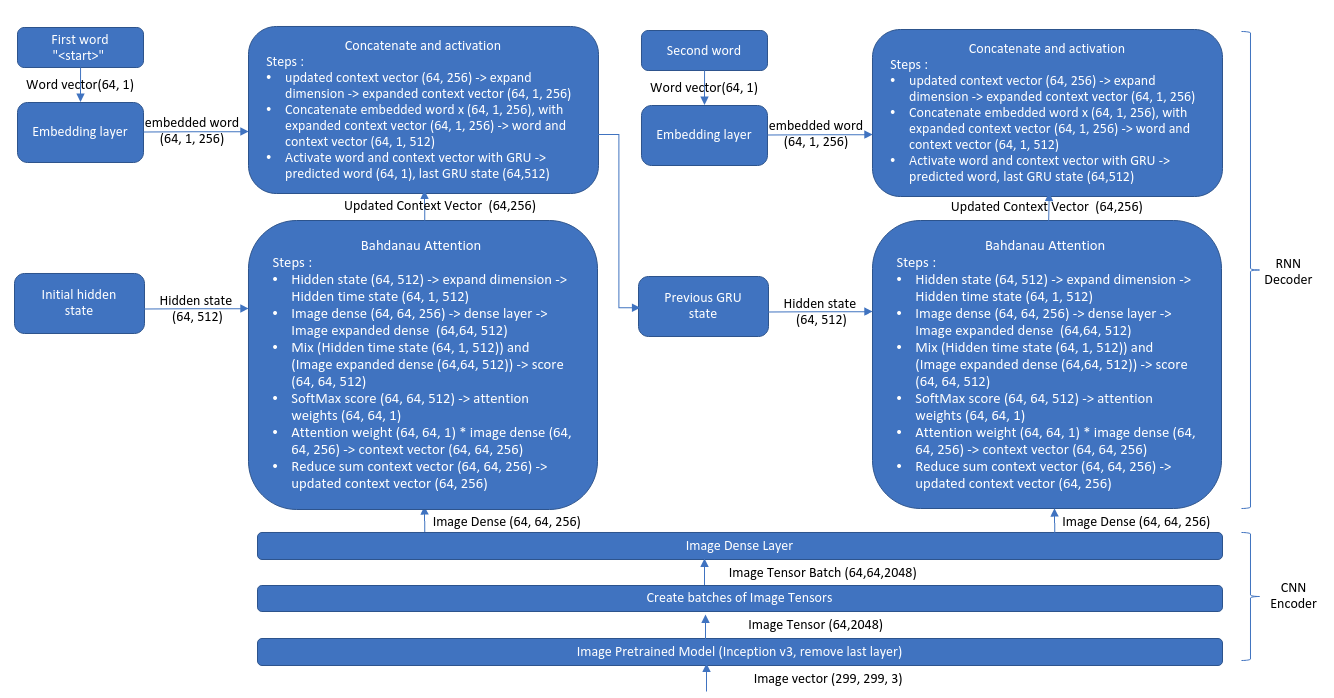Figure 1: Training Processes for Image Captioning Architecture and Flow of Tensors

Recently, I have upgraded my server from tensorflow 1 to tensorflow 2 (TF2) after knowing that tensorflow 2 has finally integrated Keras into its’ library. To get myself familiar with the new libraries, I have tested the Image captioning tutorial and found out that this tutorial is really easy to understand. Not only the codes are well-written, the provided explanations are clear as well.

However, we need to have some understandings on sequence-to-sequence (seq-to-seq) model and tensor operations to fully understand the entire flows. Therefore, I create this blog to fill-in the knowledge gap missing in the provided tutorial.

First, let’s start with some easy steps in CNN encoder. Basically, in the encoder, we will convert an image into image tensor. Then, then image tensor will be converted into a batch of image tensors.

Any image is composed of pixels with 3 channels (RGB) and we can represent the image in vector form of (x location, y location, RGB). The image vector will be the input to CNN encoder. Now, we are entering into the interesting part of CNN encoder in which transfer learning concept will be introduced.

Transfer learning means that we will borrow the pre-trained models from others and plug the model into our model. In this CNN encoder case, we will use Inception V3 pre-trained model to produce image tensor from image vector. How can we achieve that? And why we need image tensor?

`image_model = tf.keras.applications.InceptionV3(include_top=False,                                                weights='imagenet')new_input = image_model.input # (299, 299, 3)hidden_layer = image_model.layers[-1].outputimage_features_extract_model = tf.keras.Model(new_input, hidden_layer)`

We set the include_top parameter to false means that we do not want the last prediction layer of inception v3 model. Instead, we would like to use the hidden layer before the prediction layer to produce the image tensor. The image tensor produced by inception v3 model carries the image information or features known by the model. For example, when we see a man running in an image, the model needs to encode “the man” to a number and “running” action to another number. These numbers(“the man”, “running”) only know by inception v3 model but we borrow the numbers(“the man”, “running”) from Inception v3 model and integrated the numbers into our image captioning model. In general, our model is learning the meaning of number combinations (tensor) generated from the Inception v3 model.

Once we have the image tensor, we would like to create batch of image tensor. Here, we are exploiting the parallel processing from GPU. Instead of generating the image tensor(64, 2048) for one image, we would like to generate image tensors for several images. In our case, we would like to generate 64 image tensors (64, 64, 2048) at once. To visualize this, we could think that the first 64 represents 64 images, the second 64 represents different kind of man (old man, young man, angry man, mad man, spider man, etc) and the last 2048 as different kind of actions (running fast, running quickly, running slow, walking , sleeping, climbing, etc). Then, we would like to compressed the image tensors from (64, 64, 2048) to (64, 64, 256) using dense layer. For our visualization, we can imaging that this dense layer tries to combine few actions like running fast, running quickly and running slow into running. Once we have these image tensors, we are ready to go to decoder part. And this is where the magic begins.

The big picture of decoder is, we will take the previous image information and previous word information and current image information to make prediction for current word.

Without further ado, let’s dig into the details of decoder. In decoder part, we will create a loop. For each time of the loop, we take in previous image (p_image) information, previous word (p_word) information, current image information (c_image) and predict current one word (c_word). Please take note that, for the first time, an empty tensor of (64, 512) is created to represent p_image and p_word. And for the first time, p_word always starts with “<start>”. For the second time onward, (64, 512) tensors will be produced from (c_image, c_word) and c_image, c_word will be updated. This loop will end when the predicted current word is “<end>”.

`class BahdanauAttention(tf.keras.Model):  def __init__(self, units):    super(BahdanauAttention, self).__init__()    self.W1 = tf.keras.layers.Dense(units)    self.W2 = tf.keras.layers.Dense(units)    self.V = tf.keras.layers.Dense(1)# features = current image tensors # hidden = previous image and word tensors  def call(self, features, hidden):        hidden_with_time_axis = tf.expand_dims(hidden, 1)    score = tf.nn.tanh(self.W1(features) + self.W2(hidden_with_time_axis))    # which part of the image should I focus on (Attend)    attention_weights = tf.nn.softmax(self.V(score), axis=1)    context_vector = attention_weights * features    context_vector = tf.reduce_sum(context_vector, axis=1)    # context_vector (64, 256)    return context_vector, attention_weights`

Let’s start with Bahdanau Attention layer. The current batch of image tensors (64, 64, 256) will flow to Bahdanau Attention layer. This layer also takes in previous image information and word information and both information are represented in tensors of shape (64, 512). As we can see, the dimension of the current image tensors (64, 64, 256) is different with previous image and word tensors (64, 512). In order to mix these 2 tensors, we need to reshape the 2 tensors to have the same dimension. For current image tensors, we expand the image tensors from (64, 64, 256) to (64, 64, 512) using dense layer and for previous image and word tensors(64, 512), we expand the dimension to (64, 1, 512). Now, we can add them together to create mixture tensors. (64, 64, 512) + (64, 1, 512) = (64, 64, 512). Once we have the mixture tensors (64, 64, 512) of previous word, previous image information and current image information, we will perform softmax operation to find the important tensor to focus on. After softmax mixture tensors (64, 64, 512), the new tensor shape would be (64, 64, 1). The next step would be multiplying the new tensor (64, 64, 1) with current image tensor (64, 64, 256) to know the focus of the image which is known as context vector. To visualize this, we could think that the previous predicted word as “a” and the previous image information could be a human face. Then, with all the provided information(previous (text + image information)+current image information), the model could make the decision to focus on “hair” part of the image to make the word prediction. This focus process is known as attention (`attention_weights = tf.nn.softmax(self.V(score), axis=1)`) and the image part selection is done by multiplying the attention weights with current image tensors (`context_vector = attention_weights * features`). The last step in Bahdanau Attention layer is to reduce the dimension of context vector from (64, 64, 256) to (64, 256). We can call this as updated context vector and the purpose of this dimension reduction is to concatenate with the word information later.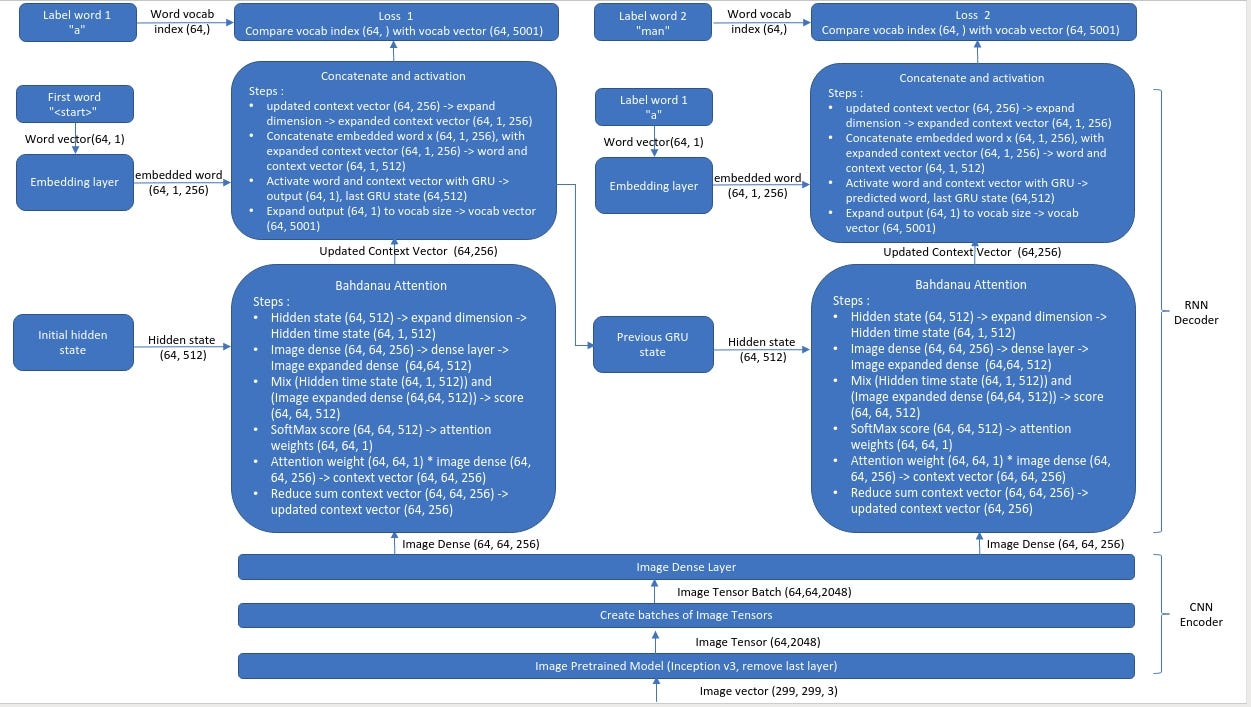Figure 2 : Image captioning architecture with labelled words and loss

Till now, we know that the model already figures out which part of the image to focus on. To increase the accuracy of the prediction for current word, we need a strong word feature from previous labelled word (64, 1). As we have known earlier, the previous labelled word would be “<start>” for the first time. During training, we will create an embedding layer for the previous labelled word. This layer actually acts like a one-hot embedding for word. When the labelled word (64, 1) flow through the embedding layer, the labelled word would become labelled word embedding(64, 1, 256). Remember that we have an updated context vector (64, 256). To concatenate the 2 tensors, we expand the dimension for the updated context vector from (64, 256) to (64, 1, 256). When the labelled word embedding(64,1,256) and the update context vector (64, 1, 256) has the same dimension, we could concatenate the embedding layers. After the concatenation, we have a new tensor of (64, 1, 512) with information of the updated context vector and previous labelled word. Let’s call this tensor (64, 1, 512)as knowledge tensor. Then, we will pass this knowledge tensor (64, 1, 512) to GRU layer where the GRU layer will update each of the 512 features with “tanh” activation function based the previous knowledge tensors. After that, it will return an output of (64, 1, 512) and the GRU layer last state (64, 512). This last state tensor will become the previous image information and word information for the next state while the output (64, 1, 512) will be passed to a dense layer of vocab size (64, 1, 5001). The purpose of this is to find out the most suitable word from the 5001 vocabs as current word prediction. The last step would be calculating the loss between the current predicted word and current labelled word.

Finally, we have gone through all the processes in the decoder layer.

I would like to show the result from my trained model for this picture.
Prediction Caption: a man in a wetsuit surfing in the ocean <end>

I train the model on my laptop with all the pictures by commenting out these few lines.

`# Select the first 30000 captions from the shuffled set# num_examples = 30000# train_captions = train_captions[:num_examples]# img_name_vector = img_name_vector[:num_examples]`

And I run the training steps using docker because I don’t want to mess up my laptop with all the libraries and dependencies. If you would like to setup nvidia-docker, tensorflow 2 and Cudnn 7, feel free to visit my another blog.

The tensorflow tutorial is provided here. if your are interested in the details, you could refer to this paper “Show, Attend and Tell: Neural Image Caption Generation with Visual Attention”. I feel that this tutorial is very fun and strongly encourage you to try. When you have doubts in the tutorial, feel free to revisit this blog. See you again…

--

--

--

## More from Machine Growth

Angel is hiding in the details

## The NOT definitive guide to learning math for machine learning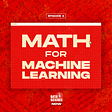## Detecting Breast Cancer with Deep Learning## Sage Advice — Deploying Machine Learning models with Amazon SageMaker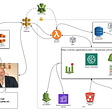## How I Got Started With Kaggle Competitions (It’s Not That Hard)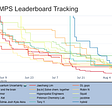## Generate stories using RNNs |Pure Mathematics with code|## Multi-GPU training with Keras, Python, and deep learning on Onepanel.io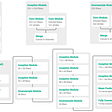## Auto Update Model’s Hyper-Parameters using DevOps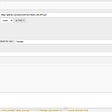## How to load COCO Keypoint Data with FiftyOne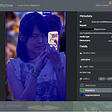## Review of Deep Learning Architectures for Image Classification Problem (Part 3)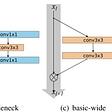## ByteBridge Launches 2D-3D Sensor Fusion Labeling Service, Providing Scalable Training Data for…## The implementation and Analysis of the Girvan-Newman Algorithm Part 1